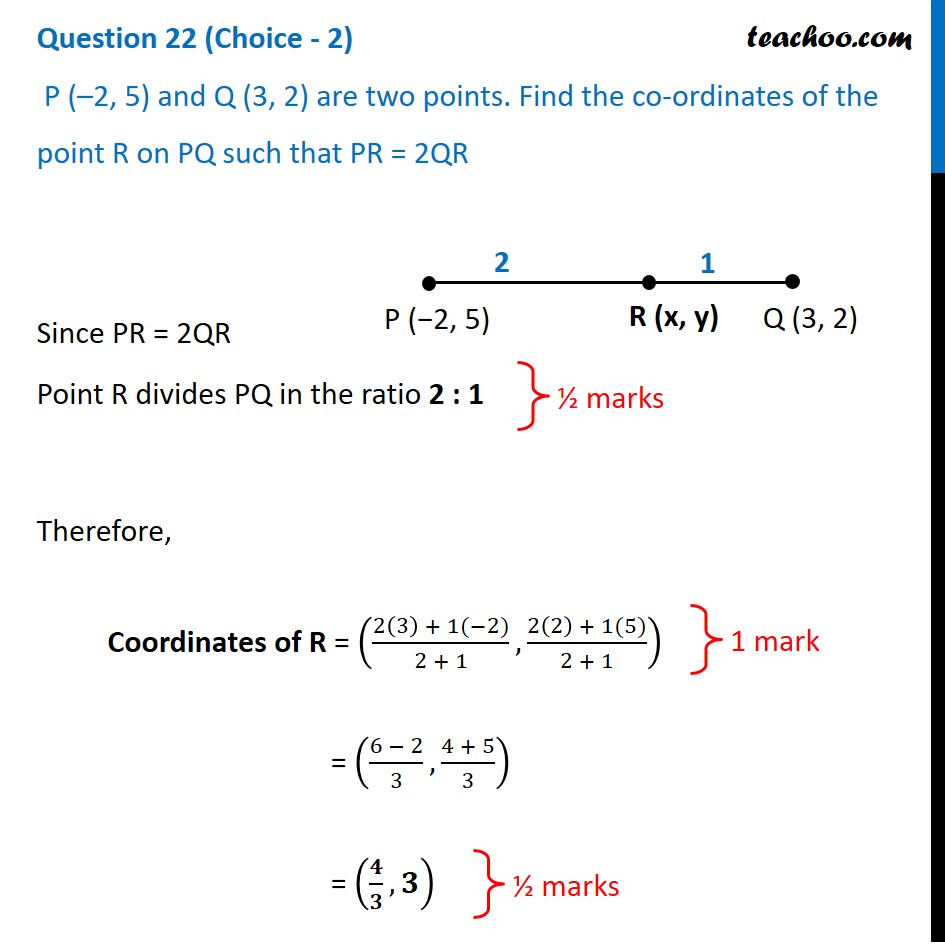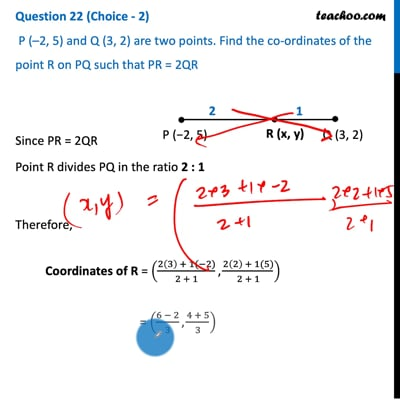CBSE Class 10 Sample Paper for 2021 Boards - Maths Standard

Class 10
Solutions of Sample Papers for Class 10 Boards

## P (–2, 5) and Q (3, 2) are two points. Find the co-ordinates of the point R on PQ such that PR = 2QRThis video is only available for Teachoo black users

Introducing your new favourite teacher - Teachoo Black, at only ₹83 per month

### Transcript

Question 22 (Choice - 2) P (–2, 5) and Q (3, 2) are two points. Find the co-ordinates of the point R on PQ such that PR = 2QR Since PR = 2QR Point R divides PQ in the ratio 2 : 1 Therefore, Coordinates of R = ((2(3) + 1(−2))/(2 + 1),(2(2) + 1(5))/(2 + 1)) = ((6 − 2)/3,(4 + 5)/3) = (𝟒/𝟑,𝟑)